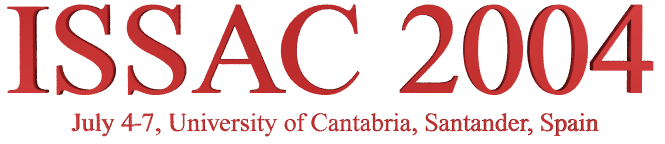General  Information
Program
Registration
Call  For
Local  Information
Miscellaneous

# Invited Talks

The following invited talks will be presented on ISSAC 2004

1. Numerical Algebraic Geometry and Symbolic Computation by Jan Verschelde
2. Triangulations of Polytopes and Algebraic Geometry by Francisco Santos
3. Sum of Squares of Polynomials and Their Applications by Pablo Parrilo

### Numerical Algebraic Geometry and Symbolic ComputationJan Verschelde University of Illinois at Chicago

In a recent joint work with Andrew Sommese and Charles Wampler, numerical homotopy continuation methods have been developed to deal with positive dimensional solution sets of polynomial systems. As solving polynomial systems is such a fundamental problem, connections with recent research in symbolic computation are not hard to find. We will address two such connections.

One part of the numerical output of our methods consists of a "membership test" used to determine whether a point lies on a positive dimensional solution component. While Gröbner bases provide an exact answer to the ideal membership test, geometrical results can be obtained at a lower complexity, as shown by Giusti and Heintz. The recent work of Grégoire Lecerf implements an irreducible decomposition in a symbolic manner.

The factorization of multivariate polynomials with approximate coefficients was posed as an open problem in symbolic computation by Erich Kaltofen. Providing a certificate for a numerical factorization by means of the linear trace is related to ideas of André Galligo and David Rupprecht, which also appears in the works of Tateaki Sasaki and collaborators.

### Triangulations of Polytopes and Algebraic GeometryFrancisco Santos University of Cantabria

The interaction between polyhedral combinatorics and algebraic geometry is a classical theme. Two examples from the 70's, in which each discipline has benefited from the other, are the algebraic proof by Stanley of the Upper Bound Theorem for polytopes and the Bernstein-Kouchnirenko-Khovanski Theorem on the number of roots of a generic sparse system via mixed volumes.

More specifically, triangulations of polytopes and point sets have algebraic-geometric applications in several contexts. Perhaps the most celebrated one is the method of Viro for the construction of real algebraic hypersurfaces with "prescribed" topology. After reviewing this and other well-known applications we will discuss in some more detail the following two recent ones in which the author has been involved: the construction of non-connected toric Hilbert schemes via triangulations of a certain 5-dimensional polytope, and the study of "tropical algebraic geometry" via triangulations of the product of two simplices.

### Sum of Squares of Polynomials and Their ApplicationsPablo Parrilo ETH Zurich

The existence of a decomposition of a multivariate polynomial as a sum of squares is a basic question in applied mathematics. It is also one with important practical consequences, as such decompositions can be used in the formulation of easily verifiable certificates of the emptiness of semialgebraic sets. Particularly exciting is the recent availability of efficient techniques, based on convex optimization, for its effective computation. In this talk we present an overview of this novel research area, that combines symbolic and computational techniques arising from real algebra and numerical optimization.

Along the way, we'll learn how to compute sum of squares decompositions for polynomials using semidefinite programming, how to certify the results using rounding techniques, and how to obtain structured infeasibility certificates for real solutions of systems of polynomial equations and inequalities. The developed techniques, based on results from real algebraic geometry, unify and generalize many well-known existing methods. The ideas and algorithms will be illustrated with examples drawn from a broad range of domains, including geometric theorem proving and quantum mechanics.

issac2004 @ risc.uni-linz.ac.at